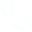# How Python Language Models with MindOpt

This article is the second in a series of articles. I will share my personal definition of mixed integer linear programming, and then give an example. Finally, I will use the MindOpt Python language API to model and solve the problems in the mixed integer linear programming problem example and show the solution results.

MindOpt Python, C, C++language solving LP, MILP, QP problem series

• Python: LP problem of linear programming, MILP problem of mixed integer linear programming (this chapter), QP problem of quadratic programming

• C: LP problem of linear programming, MILP problem of mixed integer linear programming, QP problem of quadratic programming

• C++: LP problem of linear programming, MILP problem of mixed integer linear programming, QP problem of quadratic programming

install

Users can download and install MindOpt optimization solver here, free of charge. The installation step cannot be found here.

Mixed integer linear programming

Personally, I think the difference between mixed integer linear programming and linear programming is that when solving the optimal value of the objective function in linear programming, the decision variables are continuous, that is, they can be integers or decimals. However, mixed integer linear programming may have one or more variables that must be integers.

For example, the classic backpack problem: there is a group of items on the table, each item has its own value and weight, but the weight of the bag is limited; What items should we put in the bag? Within the weight range of the bag, the total value of the items in the bag is the highest? The selected item in this item is an integer, which cannot be cut by a decimal. For example, a hair dryer cannot be cut and only half of it can be taken.

Mixed integer linear programming problem in mathematical form:

Mixed integer linear programming is more difficult to solve in many cases. When solving, branch and bound method, cutting plane method, etc. can be used, which will be cut into subproblems to call the linear programming (LP) solution module. MindOpt also released its ability to solve mixed integer linear programming (MILP) this year. Next, I will give an example of how to use it.

example

As with the linear programming in the previous chapter, we make a hypothesis about the example of mixed linear programming problem, and regard it as a real example in life, rather than as abstract as the mathematical formula.

Suppose that the factory has one raw material, which can produce a variety of parts blanks, but there are also many ways to produce each part. Each way of producing parts can obtain the number of blanks of different parts and the required quantity of each part, but one of the parts must be an integer, then how to produce to reach the required quantity of parts, and the raw materials used are the least.

Mathematical examples

Examples of mixed integer linear programming problems:

Python+MindOpt code implementation

Several APIs used by the core are:

model = MdoModel()

model.set_ int_ attr(MDO_INT_ATTR.MIN_SENSE, 1)

x.append(model.add_var(0.0, 10.0, 1.0, None, "x0", True))

model.add_ cons(1.0, MDO_INFINITY, 1.0 * x + 1.0 * x + 2.0 * x + 3.0 * x, "c0")

model.solve_ prob()

model.display_ results()

The following is a complete example, which can be copied and saved as test_ MILP.py file.

Results of MindOpt solution

Then run the code, such as python test in the command line_ After MILP.py, the result of the solution is as follows, followed by the comment I added.

## Related Articles

• ### A detailed explanation of Hadoop core architecture HDFS

Knowledge Base Team

• ### What Does IOT Mean

Knowledge Base Team

• ### 6 Optional Technologies for Data Storage

Knowledge Base Team

• ### What Is Blockchain Technology

Knowledge Base Team

## Explore More Special Offers

1. ### Short Message Service(SMS) & Mail Service

50,000 email package starts as low as USD 1.99, 120 short messages start at only USD 1.00Contact Us